GSAK (Geocaching Swiss Army Knife)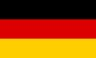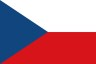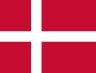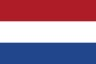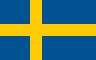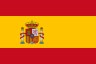Contents - Index

CsvGet (function)

CsvGet(sData,sOther) : string

This function allows you to read CSV data. It takes care of all the nuances of the standard CSV format. There are various extended definitions of CSV but the one GSAK supports is fairly standard:

 Fields are separated by commas and *optionally* enclosed in double quotes. Records are separated by CrLf.  If a field contains a comma then it must be surrounded by double quotes. If a field contains a double quote then it is escaped by using two double quotes. If a field contains a Crlf it must be surrounded by double quotes

In it's simplest form this function will parse just one line of data. However, it can also be used to parse a whole file of data.

sData - The CSV data string to parse. Use "*" to use the previous internal buffer (see notes below)
sOther - Other options that change the behavior of the function.

nn - A single number instructs the function to return the data found in that column (of the first row). Use when parsing just one line of CSV data
nn,nn - A number followed by a , (comma) followed by another number. Use to parse many lines of CSV data (eg when reading from a file or the result of using Sqlite() with the Delim=*csv* option). The first number is the row number and the second number is the column number
rows - Use the literal "rows" to return the total number of rows (lines) in your CSV data
cols - Use the literal "cols" to return the total number of columns (fields) in your csv data
Delim= - The field delimiter for a CSV file defaults to and is usually a , (comma) Use this option if your data uses a different field delimiter.

This function has been optimized by storing sData into an internal buffer, then allowing for retrieval from the internal buffer rather than suffering the overhead of replacing the buffer each time. So if sData will be the same for subsequent calls to this function, use the reserved value "*" to tell the function to use the internal buffer.

For example, you have a CSV file on disk. You want to process it, and do something with just the 3rd column in each line of the data.

 \$data = GetFile("c:\temp\test.csv") \$rows = Val(CsvGet(\$data,"rows")) \$x = 0 Repeat   \$x = \$x + 1   \$col3 = CsvGet("*","\$x,3")   // do what ever you want with \$col3 Until \$x = \$rows

Alpha List         Category List